C.I. Calculator: Diagnostic Statistics

Data Input: (Help) (Formula) (Example)

 Disease No Disease Totals Test Outcome Positive Test Outcome Negative Totals 1-α

 Result Point Estimate Lower C.I. Upper C.I. Sensitivity Specificity Positive Predictive Value Negative Predictive Value

 Diagnostic Test Point Estimate Lower C.I. Upper C.I. Pre-test probability Likelihood Ratio Positive Positive Post-test probability Likelihood Ratio Negative Negative Post-test probability

Help Aids Top

Description:

Sensitivity is the ability of the test to pick up what it is testing for and Specificity is ability to reject what it is not testing for.

Likelihood ratios determine how the test result changes the probability of certain outcomes and events.

Pre-test and Post-test probabilities are the subjective probabilities of the presence of a clinical event or status before and after the diagnostic test.

For positive test, we find the positive post-test probability and for negative test, we find the negative post-test probability.

Procedure:

1. Enter

a)    Value of Disease and No Disease in the Positive and Negative Test Outcome group

b)    Value of 1-α, the two-sided confidence level

1. Click the button “Calculate” to obtain

a)    The Sensitivity and the corresponding 100(1-α)% confidence interval

b)    The Specificity and the corresponding 100(1-α)% confidence interval

c)    The Positive Predictive Value and the corresponding 100(1-α)% confidence interval

d)    The Negative Predictive Value and the corresponding 100(1-α)% confidence interval

e)    The Pre-test probability, Positive Post-test probability, Negative Post-test probability

f)     The Likelihood Ratio Positive, Likelihood Ratio Negative and their corresponding 100(1-α)% confidence interval

1. Click the button “Reset” for another new calculation

Formula:

Variables:

 Disease No disease Totals Test Outcome Positive a (True Positive) b (False Positive) n1=a+b Test Outcome Negative c (False Negative) d (True Negative) n2=c+d Totals m1=a+c m2=b+d N=n1+n2

Top

For Sensitivity,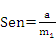Define: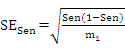The 100(1-α)% confidence interval is defined as: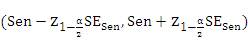For Specificity,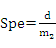Define: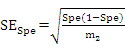The 100(1-α)% confidence interval is defined as: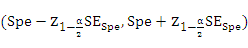For Positive Predictive Value (PPV),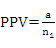Define: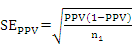The 100(1-α)% confidence interval is defined as: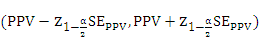For Negative Predictive Value (NPV),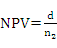Define: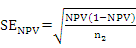The 100(1-α)% confidence interval is defined as: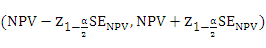Top

For Pre-test probability,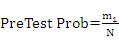For Likelihood Ratio Positive (LR+),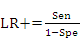Define: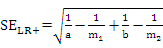The 100(1-α)% confidence interval is defined as: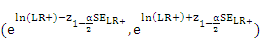For Positive Post-test probability,

Define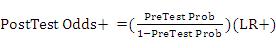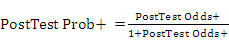For Likelihood Ratio Negative (LR-),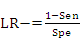Define: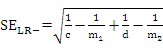The 100(1-α)% confidence interval is defined as: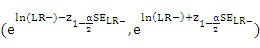For Negative Post-test probability,

Define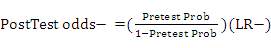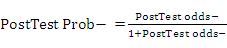Notation:

100(1-α)% confidence interval: We are 100(1-α)% sure the true value of the parameter is included in the confidence interval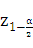: The z-value for standard normal distribution with left-tail probability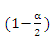Example

Suppose

 Disease No disease Totals Test Outcome Positive a=20 b=180 n1=200 Test Outcome Negative c=10 d=1820 n2=1830 Totals m1=30 m2=2000 N=2030

Then the Sensitivity is 0.66667 and the corresponding 95% C.I. ((1-α) =0.95) is (0.49798, 0.83535).

The Specificity is 0.91 and the 95% C.I. is (0.89746, 0.92254).

The Positive Predictive Value (PPV) is 0.1 and the 95% C.I. is (0.05842, 0.14158).

The Negative Predictive Value (NPV) is 0.99454 and the 95% C.I. is (0.99116, 0.99791).

The Pre-Test Probability is 0.01478.

The Likelihood Ratio Positive (LR+) is 7.40741 and the 95% C.I. is (5.54896, 9.88828).

The Positive Post-Test Probability is 0.1.

The Likelihood Ratio Negative (LR-) is 0.3663 and the 95% C.I. is (0.22079, 0.60771).

The Negative Post-Test Probability is 0.00546.

Top